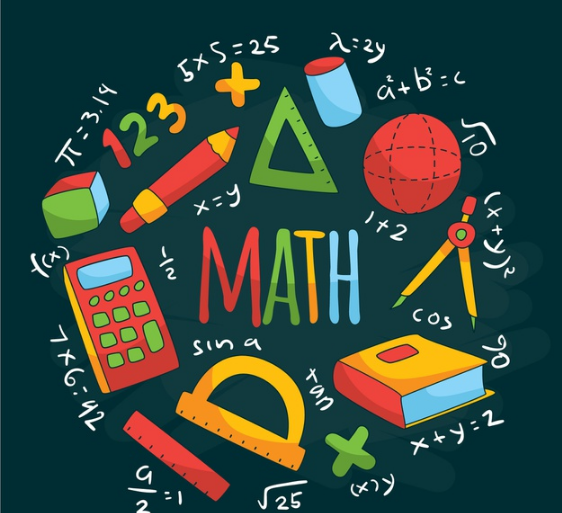## CBSE Class 6 Maths Notes Chapter 4 Basic Geometrical Ideas

### Introduction to Basic Geometrical Ideas

In this chapter, several important geometric terms.The topics that are introduced not only will help students to build a foundation in geometry but will also help them to grasp the higher-level concepts in the later grades easily.Basic Geometrical Ideas Class 6 Notes Maths Chapter 4

The main topics covered here are-

• Basic Definitions in Geometry
• Polygons
• Triangles
• Quadrilaterals
• About Circles

Download Class 6 Science Notes all chapters

Download NCERT Solutions Class 6 science all chapters

Download NCERT Solutions Class 6 all subjects

Download Class 6 all subjects notes

### Exercise 4.1 –  Basic Geometrical Shapes

• The term ‘Geometry’ is the English equivalent of the Greak word ‘Geometron’.
• ‘Geo’ means Earth and ‘metron’ Means Measurement.
• Geometrical ideas are reflected in fill forms of art, measurements, architecture, engineering, etc.
• We observe and use different objects.
• These objects have different shapes.
• The ruler is straight whereas a ball is round.

### Exercise 4.2 –  Curves

• Any drawing (straight or non-straight) drawn without lifting the pencil from the paper and without the use of a ruler is called a curve.
• In everyday use the curve means ‘not straight’ but in mathematics, a curve can be a straight line also.
• A curve is called a simple curve if it does not cross itself.
• A curve is said to be a closed curve if its ends are joined; otherwise, it is said to be open.
• In a closed curve, there are three disjoint parts:
• Interior
• Boundary
• Exterior
• The interior of a curve together with its boundary is called its region.
• In simple terms, any line that is not straight is said to be a curve.
• If a curve does not cross itself, then it is called a simple curve.

### Exercise 4.3 –  Angles

• An angle is made by two rays starting at a common endpoint.
• These rays are called the arms of the angle.
• The common endpoint is called the vertex of the angle.
• While naming an angle the vertex of the angle has to be in the middle.
• For example: Ray OP and Ray OQ form an angle. It can be denoted as ∠POQ.
• Ray OP and OQ are the arms or sides of the angle. O is the vertex of the angle POQ

### Exercise 4.4 –  Triangles

• A triangle is a three-sided polygon.
• Actually, it is a polygon with the least number of sides.
• Triangle ABC is written as ∆ABC. There are three sides of a triangle.
• Thus, sides of ∆ABC are ,(overline {AB} ,overline {BC} ) and (overline {CA} ) .. There are three angles in a triangle.
• Thus, angles of ∆ABC are ∠BAC, ∠ABC, and ∠BCA.
• The points A, B, and C are called the vertices of the triangle ABC.
• Like angle, a triangle also has three regions associated with it.
• On the triangle
• The interior of the triangle
• The exterior of the triangle.

### Exercise 4.5 –  Quadrilaterals

A quadrilateral is defined as a four-sided polygon i.e. having 4 line segments or sides and thus, 4 angles. A diagram of a quadrilateral is given for better understanding. It should be noted that the vertices of a quadrilateral are named in a cyclic manner.

In this diagram, AD and DC are one of the examples of adjacent sides. Also, AD and BC are one of the opposite sides. Here, angle A and angle C are opposite angles. In this, if the line segment is drawn from A to C or from B to D, it becomes a diagonal.

### Exercise 4.6 –  Circle

• A circle is a path of a point moving at the same distance from a fixed point.
• The fixed point is called the center, the fixed distance is called the radius and the distance around the circle is called the circumference.
• A chord of a circle is a line segment joining any two points on the circumference.
• A diameter is a chord passing through the center.
• A diameter is double the size of a radius.
• Any diameter of a circle divides it into two semi-circles.
• Any portion of a circle is called an arc.
• For two points P and Q on the circle, we get the arc PQ denoted PQ(vector)
• Like a simple closed curve, there are three regions associated with a circle.
• On the circle
• The interior of the circle
• The exterior of the circle.

Polygons

• A polygon is a closed curve made up entirely of line segments.
• The line segments forming a polygon are called its sides.
• The meeting point of a pair of sides is called its vertex.
• Any two sides with a common endpoint are called the adjacent sides.
• The endpoints of the same side are called the adjacent vertices.
• The join of any two non-adjacent vertices is called a diagonal of the polygon.

## Science Olympiad

Learn with best notes,free videos,practice questions and mock tests with School Connect Online for free demo click hereSchool Connect Online#### By School Connect Online

School Connect Online is an Integrated Learning Program for Academic Institution,and supported and mentored by StartUp Oasis,an inititive of CIIE IIM-Ahmedabad. Please visit school.schoolconnectonline.com